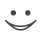This flashcard is just one of a free flashcard set. See all flashcards!

229
9.A variable x starts at zero and follows the generalized Wiener process
dx = a dt + b dz
where time is measured in years. During the first two years a=3 and b=4. During the following three years a=6 and b=3. What is the expected value of the variable at the end of 5 years
A.16
B.20
C.24
D.30